# Concurrent Execution on Simulink® Real-Time™

This example shows how to apply explicit partitioning to enhance concurrent execution of a real-time application that you generate by using Simulink Real-Time.

Simulink Real-Time supports concurrent execution by using implicit partitioning or explicit partitioning of models. This example shows the relationship between the explicit partitioning of the tasks in the model subsystems and the execution of tasks by using the Simulink Real-Time profiling tool.

The example model `slrt_ex_mds_and_tasks` runs at sample rate of 0.001 second.

To run the model with adjusted sample rate of 0.01 second, change the sample rated before running the example. In the MATLAB Command Window, type:

Ts = 0.01;

The explicit partitioning in the top-level model occurs in subsystem1.

```open_system(fullfile(matlabroot,'toolbox','slrealtime','examples','slrt_ex_mds_subsystem1')); ```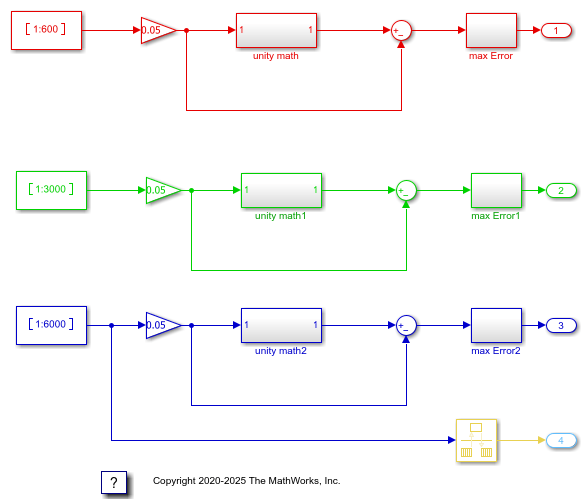The explicit partitioning in the top-level model occurs in subsystem2.

```open_system(fullfile(matlabroot,'toolbox','slrealtime','examples','slrt_ex_mds_subsystem2')); ```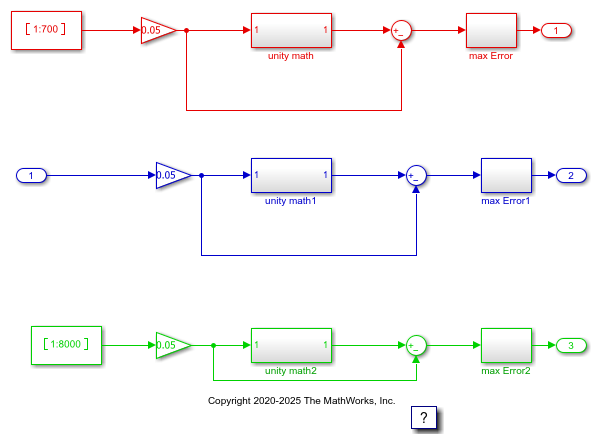Open the model `slrt_ex_mds_and_tasks`. The model is mapped to seven threads: `Model1_R1`, `Model1_R2`, `Model1_R3`, `Model1_R4`, `Model2_R1`, `Model2_R3`, and `Model2_R4`.

These threads run at sample rates of `Ts`, `2*Ts`, `3*Ts`, `4*Ts`, `Ts`, `3*Ts`, and `4*Ts`.

```model='slrt_ex_mds_and_tasks'; open_system(fullfile(matlabroot,'toolbox','slrealtime','examples',model)); ```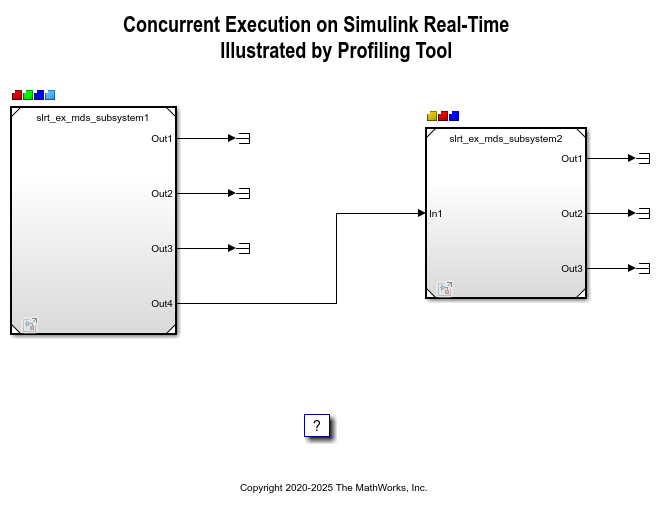To apply explicit partitioning, in the Simulink Editor, on the Real-Time tab, click Hardware Settings, and then select Solver > Configure Tasks. Select the `Tasks and Mapping` node.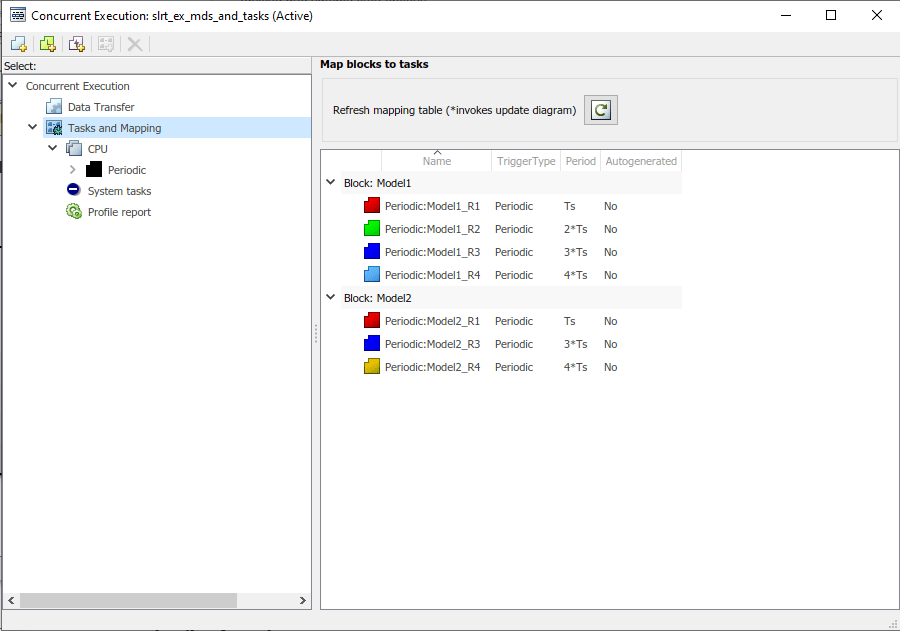```set_param(model,'RTWVerbose','off'); evalc('slbuild(model)'); tg = slrealtime; load(tg,model); % Open TET Monitor slrtTETMonitor; % Start profiler on the target computer startProfiler(tg); start(tg); pause(2); stop(tg); ```

### Display Profiling Data

The profiling data shows the execution time of each thread on a multi-core target computer.

```profData = tg.getProfilerData; profData.plot; ```
```Processing data on target computer ... Transferring data from target computer ... Processing data on host computer ... ```

### View TET Information in TET monitor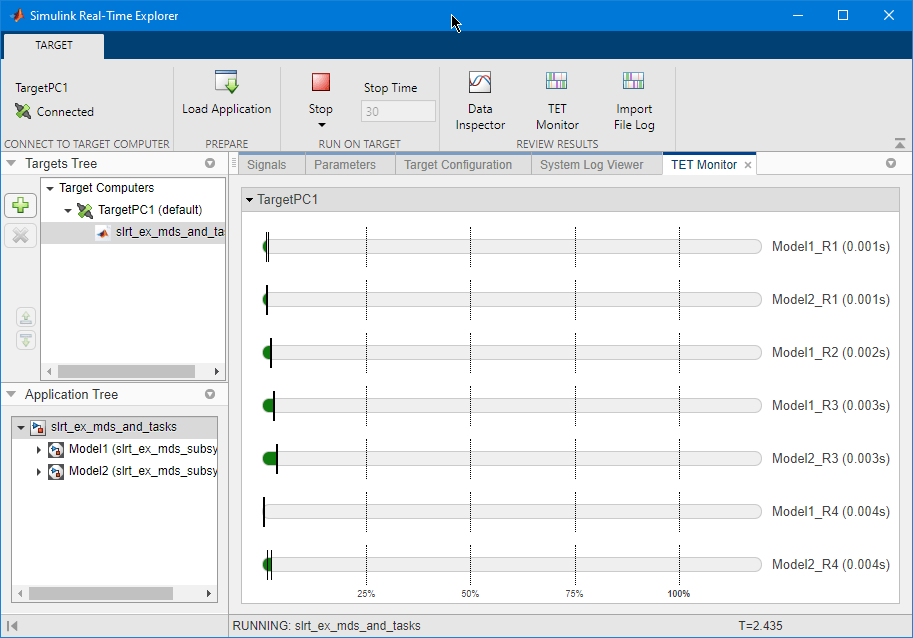### View TET Information in the Simulation Data Inspector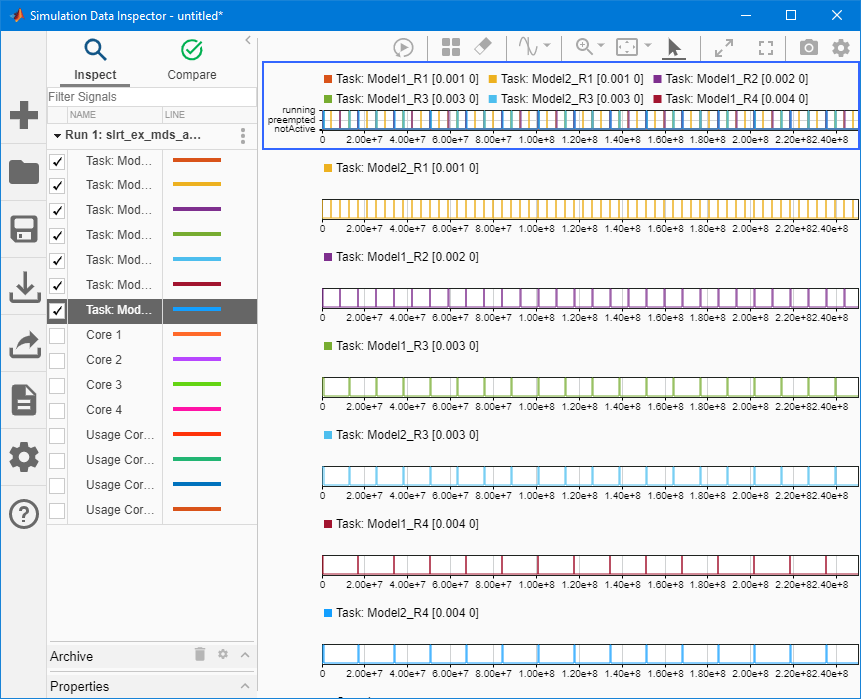```bdclose('all'); ```